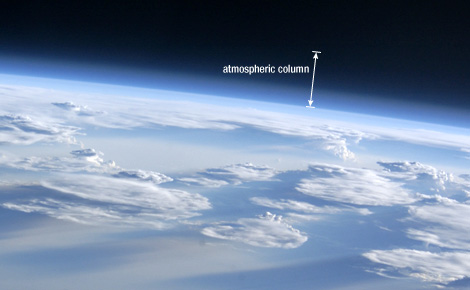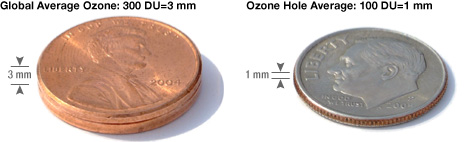Images, data, and information for the Northern Hemisphere

# What is a Dobson Unit?

The Dobson Unit is the most common unit for measuring ozone concentration. One Dobson Unit is the number of molecules of ozone that would be required to create a layer of pure ozone 0.01 millimeters thick at a temperature of 0 degrees Celsius and a pressure of 1 atmosphere (the air pressure at the surface of the Earth). Expressed another way, a column of air with an ozone concentration of 1 Dobson Unit would contain about 2.69x1016ozone molecules for every square centimeter of area at the base of the column. Over the Earth’s surface, the ozone layer’s average thickness is about 300 Dobson Units or a layer that is 3 millimeters thick.Seen from space, the edge of the Earth is blurred by the pale blue atmosphere. Most dense at the surface, the atmosphere thins with altitude, until it gradually merges with vacuum. Total ozone is measured through the entire atmospheric column, from the surface to the edge of space. (Image Science and Analysis Laboratory, NASA-Johnson Space Center. “The Gateway to Astronaut Photography of Earth.” ISS011-E-5487[07/22/2011 17:01:03])

Ozone in the atmosphere isn’t all packed into a single layer at a certain altitude above the Earth’s surface; it’s dispersed. Even the stratospheric ozone known as “the ozone layer” is not a single layer of pure ozone. It is simply a region where ozone is more common than it is at other altitudes. Satellite sensors and other ozone-measuring devices measure the total ozone concentration for an entire column of the atmosphere. The Dobson Unit is a way to describe how much ozone there would be in the column if it were all squeezed into a single layer.

The average amount of ozone in the atmosphere is roughly 300 Dobson Units, equivalent to a layer 3 millimeters (0.12 inches) thick—the height of 2 pennies stacked together. What scientists call the Antarctic Ozone “Hole” is an area where the ozone concentration drops to an average of about 100 Dobson Units. One hundred Dobson Units of ozone would form a layer only 1 millimeter thick if it were compressed into a single layer, about the height of a dime.Compressed to sea-level pressure, the ozone in the atmosphere would form a layer about the height of two pennies stacked together. Levels in the ozone hole are much less—only the height of a single dime.

How much is this, compared to the rest of the atmosphere? If all of the air in a vertical column that extends from the ground up to space were collected and squeezed together at a temperature of 0 degrees Celsius and a pressure of 1 atmosphere, that column would be 8 kilometers thick (or about 5 miles). Compare that to the 3 millimeters described above, and you may realize just how tenuous is the Earth’s ozone layer.